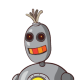## COMBAT - Air Combat

An air combat is on the way, you are asked to command this war. Now planes of enemy are full of the sky. A plane is described with three-dimensional coordinate (x, y, z)(1000<x, y, z<1200), and all coordinates are integers. As is show below:You have created a missile which can destroy all the planes in a cube whose center is (x, y, z), and the cube can be as large as (x-r, y-r, z-r) (x+r, y+r, z+r). This missile is so fierce that it will destroy not only enemy in that space, but also friends. And after that a position is occupied by a plane belongs to the opposite side before the explosion, that is to say an enemy plane will be replaced by a friend, a friend will be replaced by an enemy.

You want to reduce our loss, so you need to know which side a plane belongs to in a position.### Input

The first line contains the number of scenarios.
For each scenario you are given a line containing x1 y1 z1 x2 y2 z2, defining the two corners A(x1, y1, z1), B(x2, y2, z2) (1000<= x1<x2, y1<y2, z1<z2 <=1200) of the sky. The combat is so fierce that every point in the cube is occupied by an enemy plane at first.

Next line is a number of operation q.

Next q lines: (0<q<10000)

A character ‘U’:  followed by 4 integers, a center point M(xi, yi, zi) ,the range is ri.

A character ‘Q’:  followed by 3 integers, a position N(xi, yi, zi), if a plane belongs to us, print ”Friend” else print ”Enemy”.

Points M and N are all in cube given above.

### Output

Print a line for every ‘Q’ operation.

### Example

`Input:11000 1000 1000 1002 1002 10024U 1000 1000 1000 0U 1001 1001 1001 1Q 1000 1000 1000Q 1001 1001 1001Output:EnemyFriend`:D: 2011-01-27 07:19:38 Unfortunately this is a typical TRAP problem. The intended approach shouldn't be enough with constraints given. I guess source limit is somewhat of a hint, but that still wouldn't rule out a short but logically complicated algo.Muntasir Azam Khan: 2009-11-15 17:40:56 Mentioning the number of cases would be helpful.刘启鹏: 2009-11-15 04:34:37 the problem is so ... Re by Xilinx: The source limit tells us that. Last edit: 2009-11-15 11:03:18